# Why error message #NUM! occurs and how to solve it

Uploaded time: March 7, 2022 Difficulty: Intermediate# Why error message #NUM! occurs and how to solve it

We may encounter some errors when calculating in tables. One of these errors is #NUM!.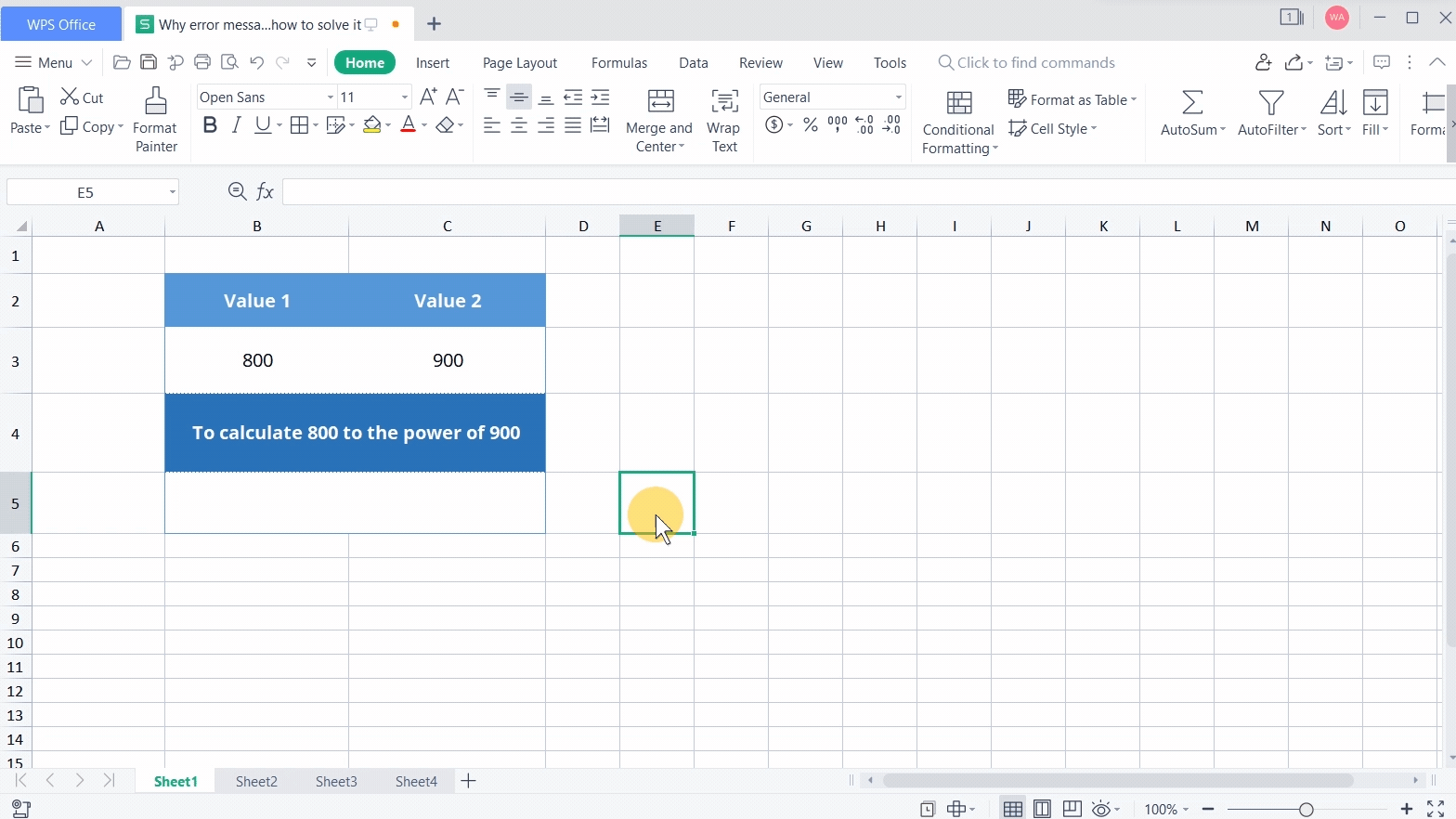There are mainly three causes:

1. The calculated value is too large or too small.

2. Invalid values are referenced in the formula.

3. The iterative function fails to calculate the result.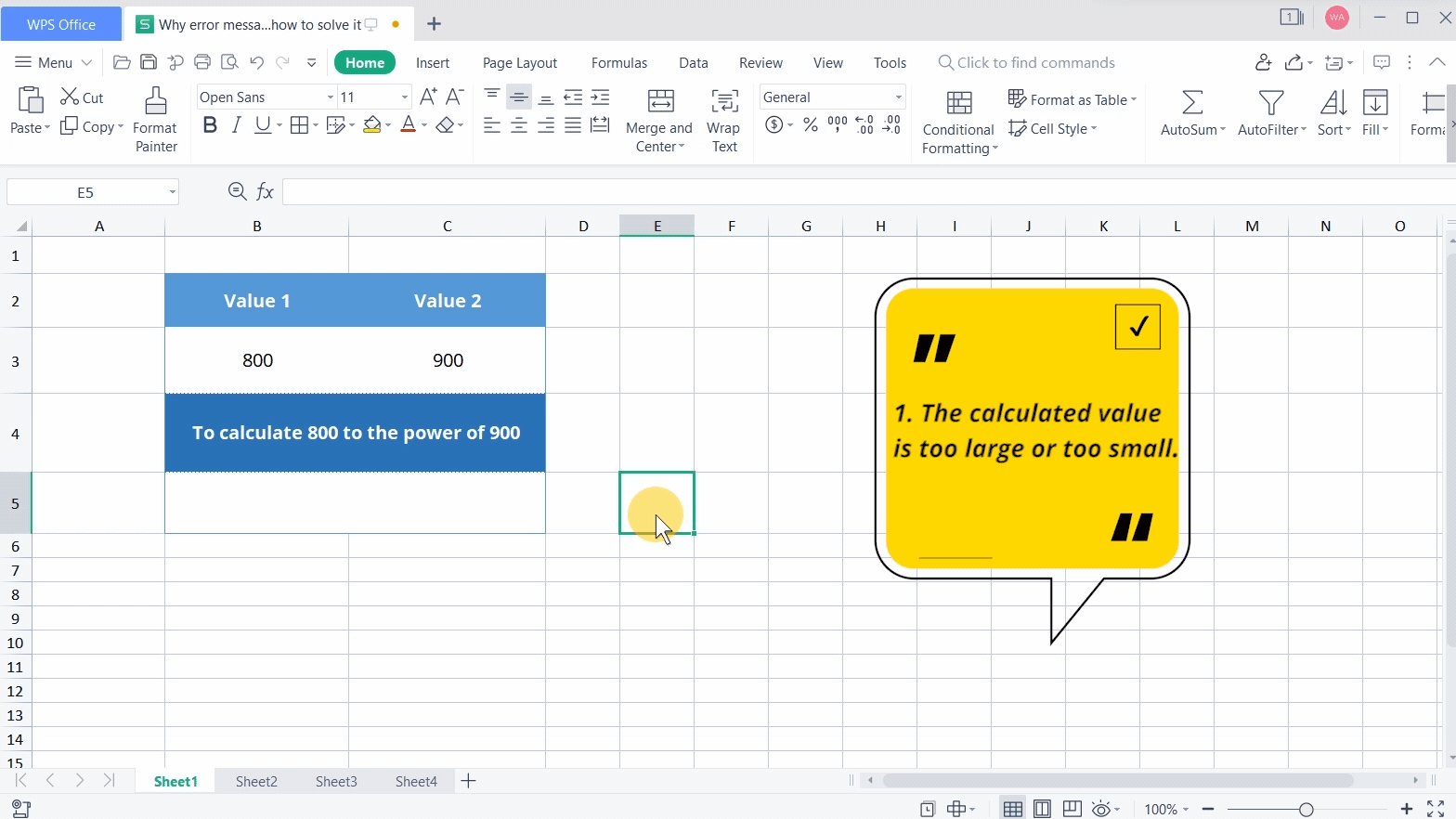· 1. The calculated value is too large or too small.

Take this table as an example. To calculate eight hundred to the power of nine hundred, input =B3^C3, which means B3 to the power of C3. Then it returns #NUM!.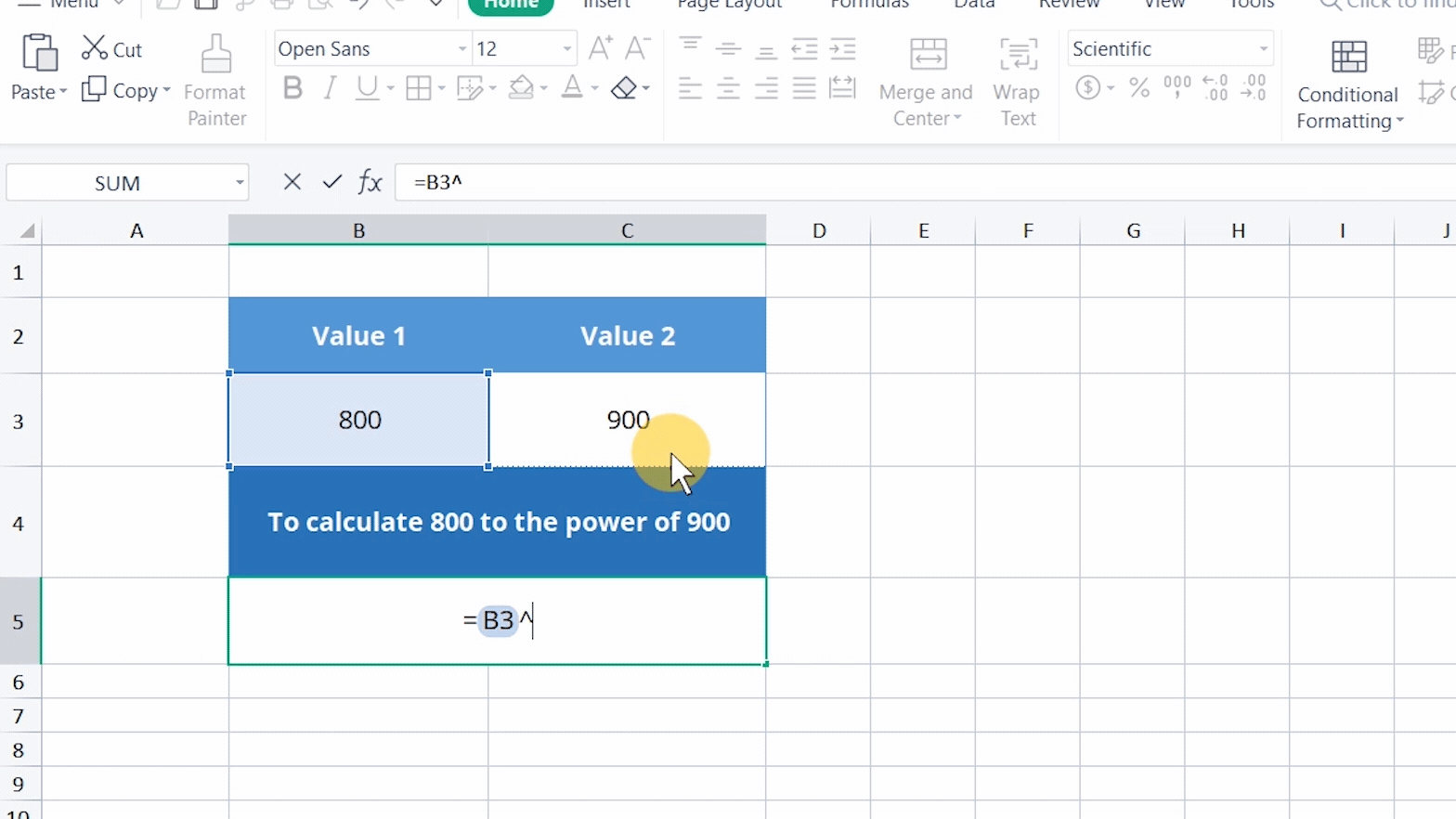That is because the calculated value is too large.

The solution is to limit the values to the computable range of the table.

· 2. Invalid values are referenced in the formula.

Take this table as an example. To calculate the square root of minus eight, input =SQRT(B3) and press the Enter key. Then it returns #NUM!.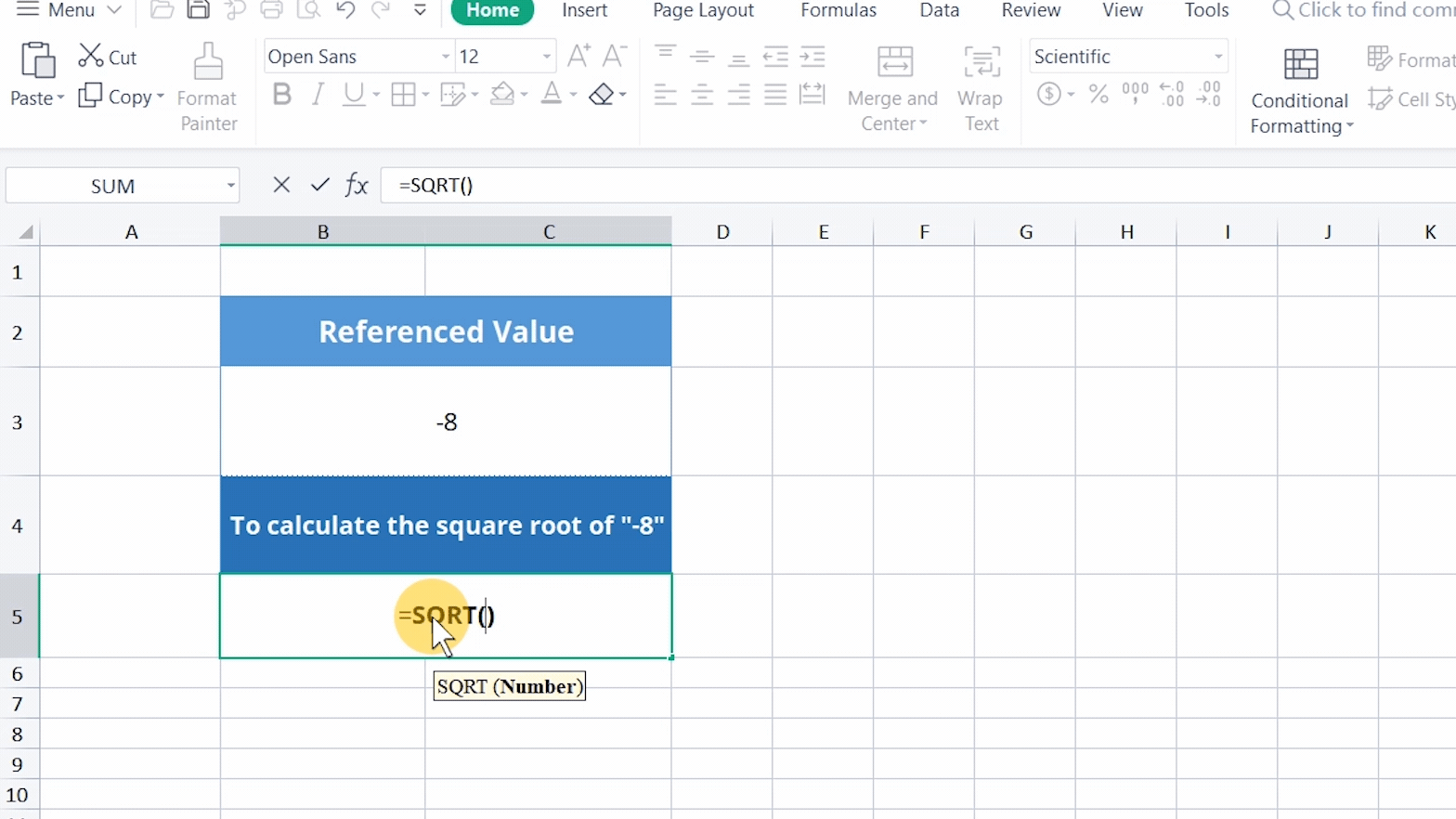That is because the value in cell B3 is negative, but you cannot take the square root of a negative value. The formula here references an invalid value.

Besides, it's easy to reference invalid values when calculating rates by the RATE function.

Take this table as an example. To calculate the monthly interest rate, input =Rate(C3*12, C4, C5). The unit of the length of maturity is the year, so we need to multiply the monthly repayment by “12” and press the Enter key. Then it returns #NUM!.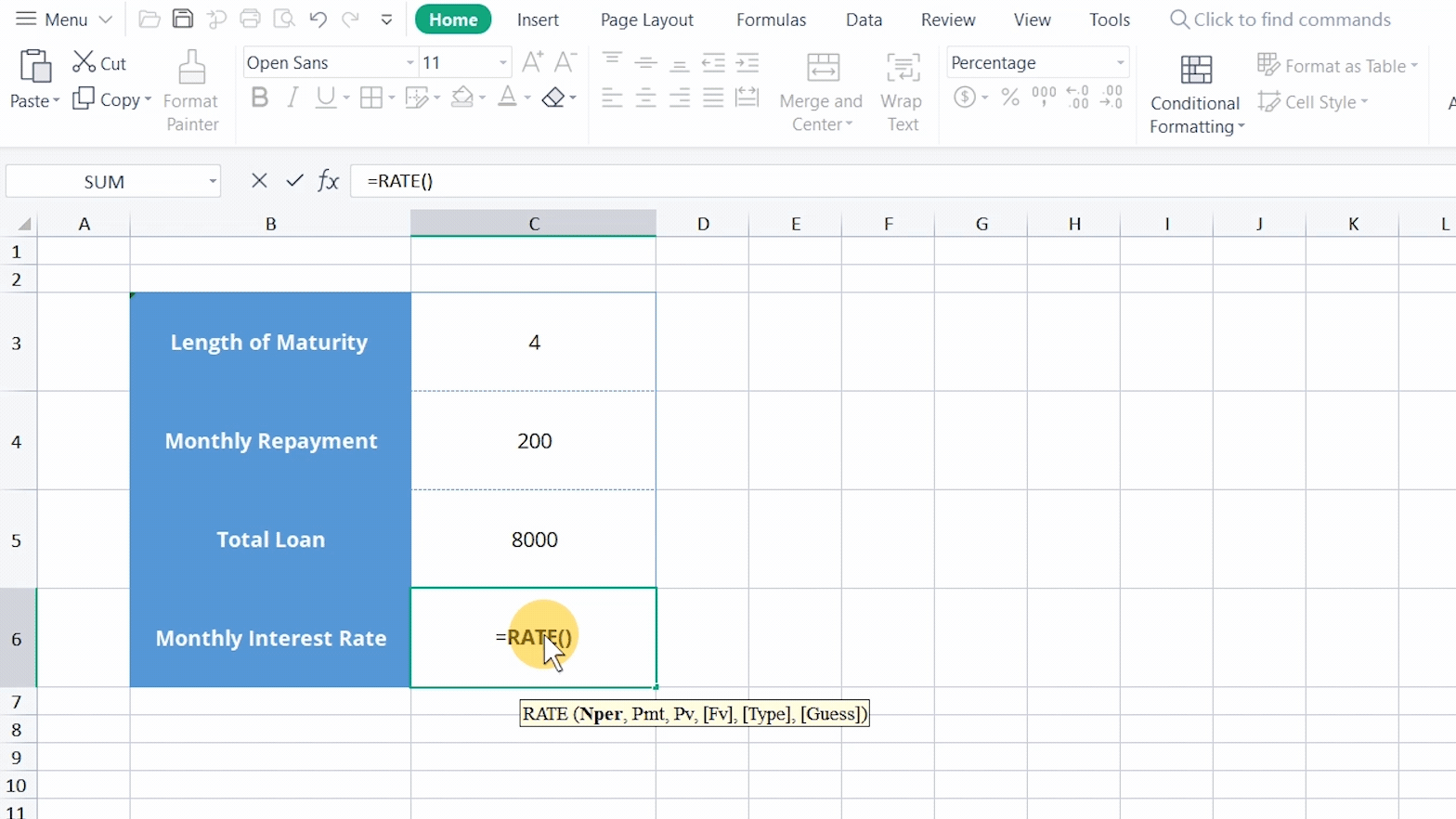That is because the value in cell C4 refers to the monthly repayment, and it is an expense, so it should be a negative value.

The solution is to add a minus sign to cell C4 to get the correct result.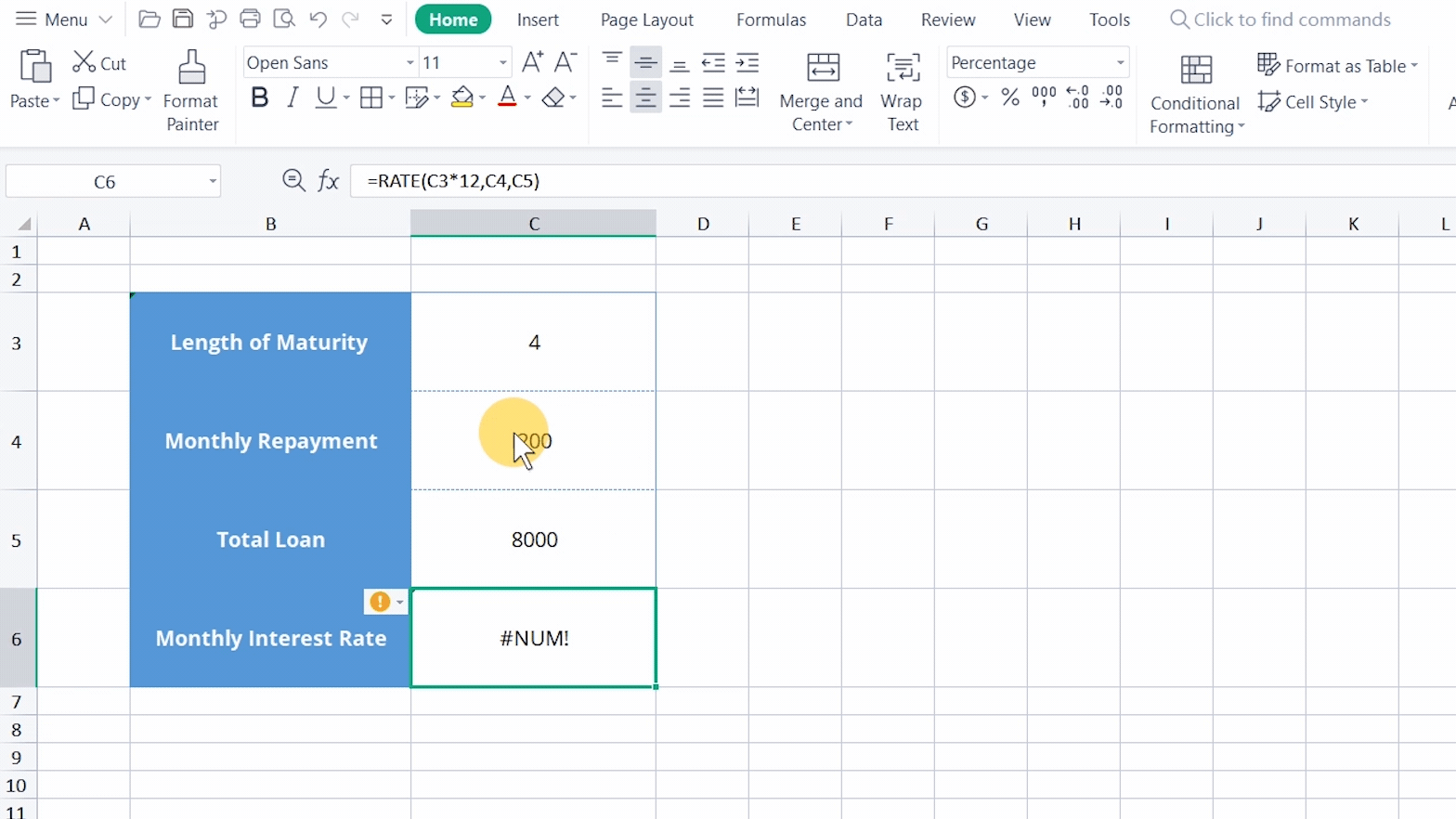· 3. The iterative function fails to calculate the result.

In addition to the above two causes, the error message #NUM! also occurs when the IRR and RATE functions go through several iterations but fail to calculate the result.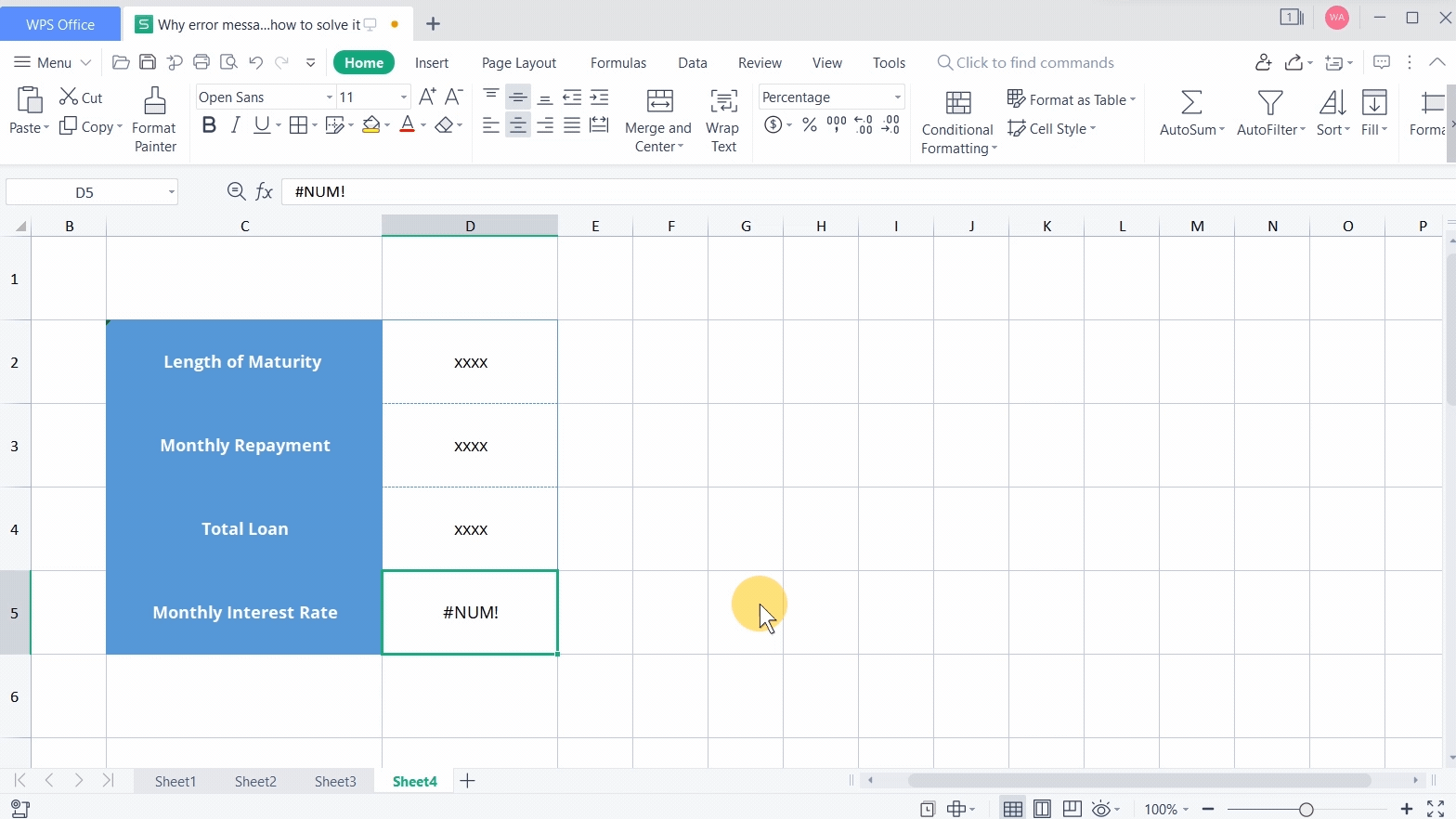The solution is to change the Maximum iterations and Maximum change.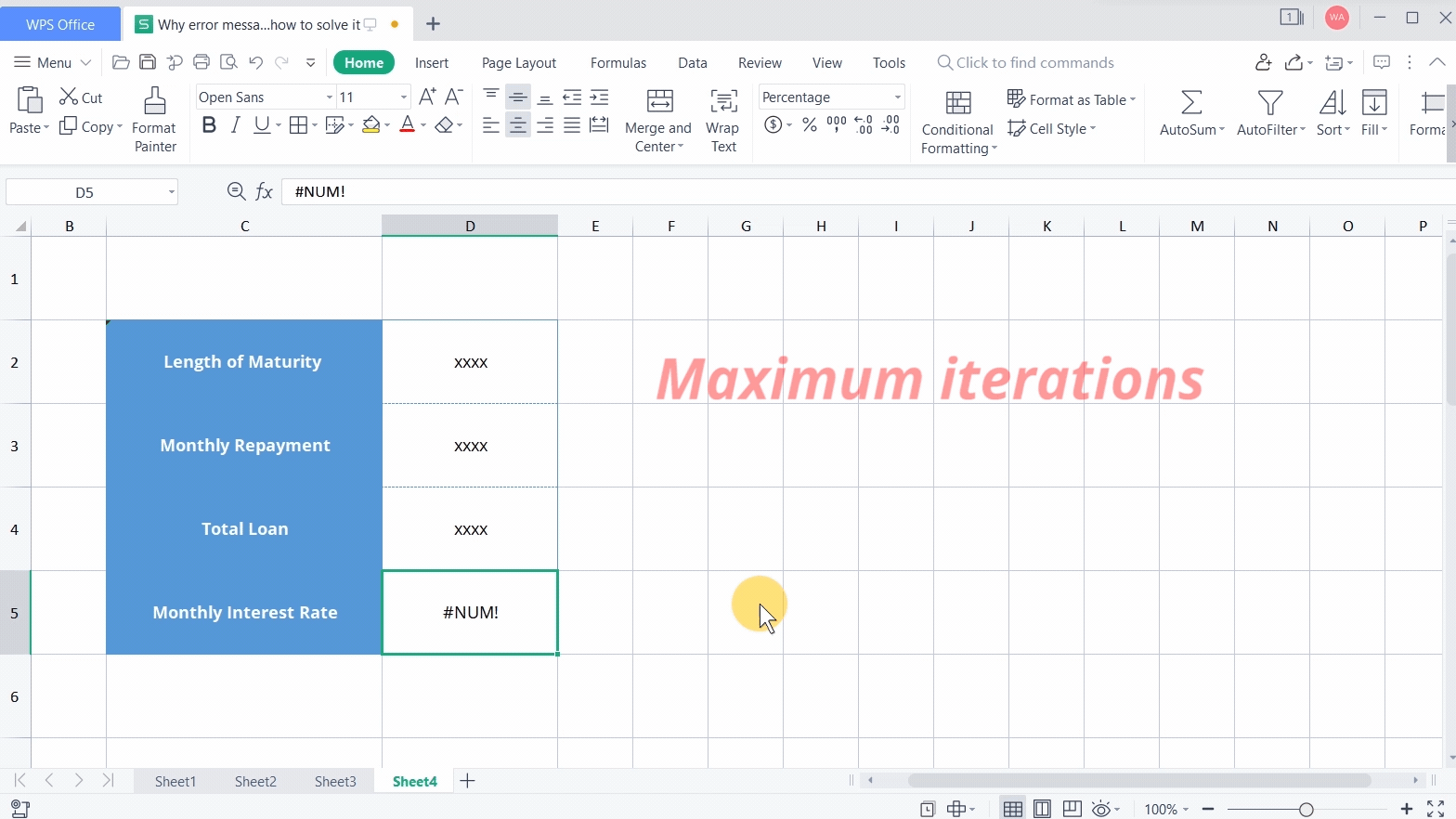Click Menu in the upper left corner, click Options, and then click Calculation. Input the expected iterations at Maximum iterations.The more iterations, the longer time it takes to calculate the result. Input the acceptable calculation error at Maximum change. The smaller the value, the more accurate the result and the longer time it takes to calculate.